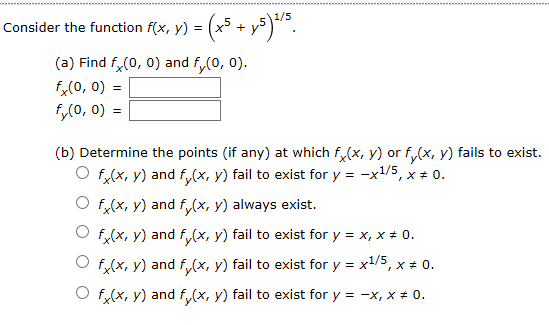# Consider the function f(x, y) =(a) Find f(0, 0) and f(0, 0)fx(0, 0)fy(o, 0)=(b) Determine the points (if any) at which f(x, y) or fy(x, y) fails to existf(x, y) and f(x, y) fail to exist for y -x1/5, x 0.O f(x, y) and f(x, y) always exist.Of(x, y) and fx, y) fail to exist for y x, x # 0f(x, y) and f(x, y) fail to exist for y x1/5, x * 0.f(x, y) and f (x, y) fail to exist for y0.-x, x

Question
9 viewshelp_outlineImage TranscriptioncloseConsider the function f(x, y) = (a) Find f(0, 0) and f(0, 0) fx(0, 0) fy(o, 0)= (b) Determine the points (if any) at which f(x, y) or fy(x, y) fails to exist f(x, y) and f(x, y) fail to exist for y -x1/5, x 0. O f(x, y) and f(x, y) always exist. Of(x, y) and fx, y) fail to exist for y x, x # 0 f(x, y) and f(x, y) fail to exist for y x1/5, x * 0. f(x, y) and f (x, y) fail to exist for y 0. -x, x fullscreen
check_circle

Step 1

According to the given information the given function is:

Step 2

For part (a)

First find fx and fy

Step 3

Now find fx(0,0) and fy(0,0):

...

### Want to see the full answer?

See Solution

#### Want to see this answer and more?

Solutions are written by subject experts who are available 24/7. Questions are typically answered within 1 hour.*

See Solution
*Response times may vary by subject and question.
Tagged in

### Calculus